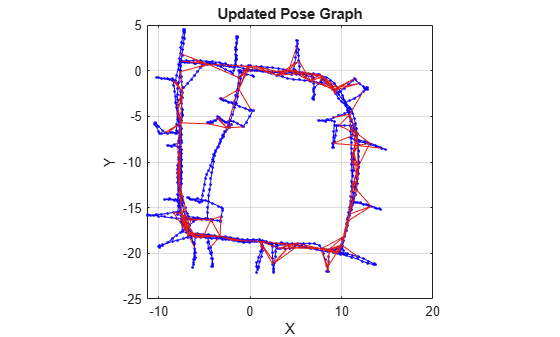# edgeNodePairs

Edge node pairs in pose graph

Since R2019b

## Syntax

``nodePairs = edgeNodePairs(poseGraph)``
``nodePairs = edgeNodePairs(poseGraph,edgeIDs)``

## Description

````nodePairs = edgeNodePairs(poseGraph)` returns all edges in the specified pose graph as a list of node ID pairs. Each row of the `edges` output is a pair of nodes that form an edge. Multiple edges may exist between the same pair of nodes.```

example

````nodePairs = edgeNodePairs(poseGraph,edgeIDs)` returns edges corresponding to the specified edge IDs. Each edge in the pose graph has a unique ID even if the node pairs are the same.```

## Examples

collapse all

This example shows how to identify and remove spurious loop closures from pose graph. To do this, you can modify the relative pose of a loop closure edge and try optimizing the pose graph with and without removing the auto spurious loop closure and compare the results.

Load the Intel Research Lab Dataset that contains a 2-D pose graph. Optimize the pose graph. Plot the pose graph with IDs off. Red lines indicate loop closures identified in the dataset.

```load intel-2d-posegraph.mat pg optimizedPG = optimizePoseGraph(pg); show(optimizedPG,IDs="off"); title("Optimized Pose Graph")```Modify the relative pose of the loop closure edge `1386` to some random values.

```loopclosureId = 1386; nodePair = edgeNodePairs(optimizedPG,loopclosureId); [relPose,infoMat] = edgeConstraints(optimizedPG,loopclosureId); relPose(2) = -5; relPose(3) = 1.5; addRelativePose(optimizedPG,relPose,infoMat,nodePair(1),nodePair(2));```

Optimize the pose graph without auto loop closure trimming. Plot the optimized pose graph to see the poor adjustment of the nodes with loop closures.

```[updatedPG,solutionInfo] = optimizePoseGraph(optimizedPG); show(updatedPG,IDs="off"); title("Updated Pose Graph")```Certain loop closures should be trimmed from the pose graph. Use the `trimLoopClosures` function to trim these bad loop closures. Set the truncation threshold and maximum iterations for the trimmer parameters.

`trimParams = struct("TruncationThreshold",0.5,"MaxIterations",100);`

Generate solver options.

`solverOptions = poseGraphSolverOptions("g2o-levenberg-marquardt");`

Use the `trimLoopClosures` function with the trimmer parameters and solver options. Plot the new pose graph to see the bad loop closures were removed.

```[newPG,trimInfo] = trimLoopClosures(updatedPG,trimParams,solverOptions); show(newPG,IDs="off"); title("New Pose Graph")```## Input Arguments

collapse all

Pose graph, specified as a `poseGraph` or `poseGraph3D` object.

Edge IDs, specified as a vector of positive integers.

## Output Arguments

collapse all

Edge node pairs in pose graph, returned as n-by-2 matrix that lists the IDs of the two nodes that each edge connects. Each row is a pair of nodes that form an edge. Multiple edges may exist between the same pair of nodes, so the matrix may contain duplicate entries.

## Version History

Introduced in R2019b

expand all